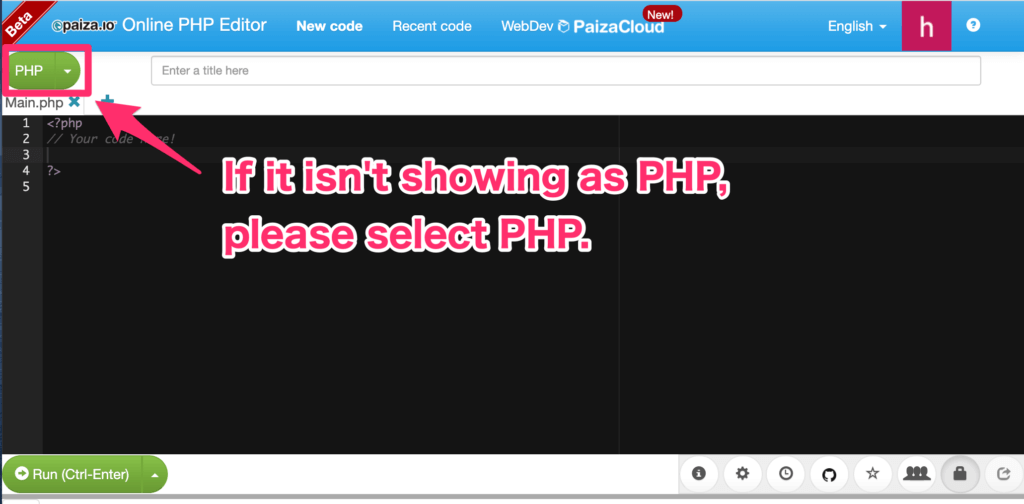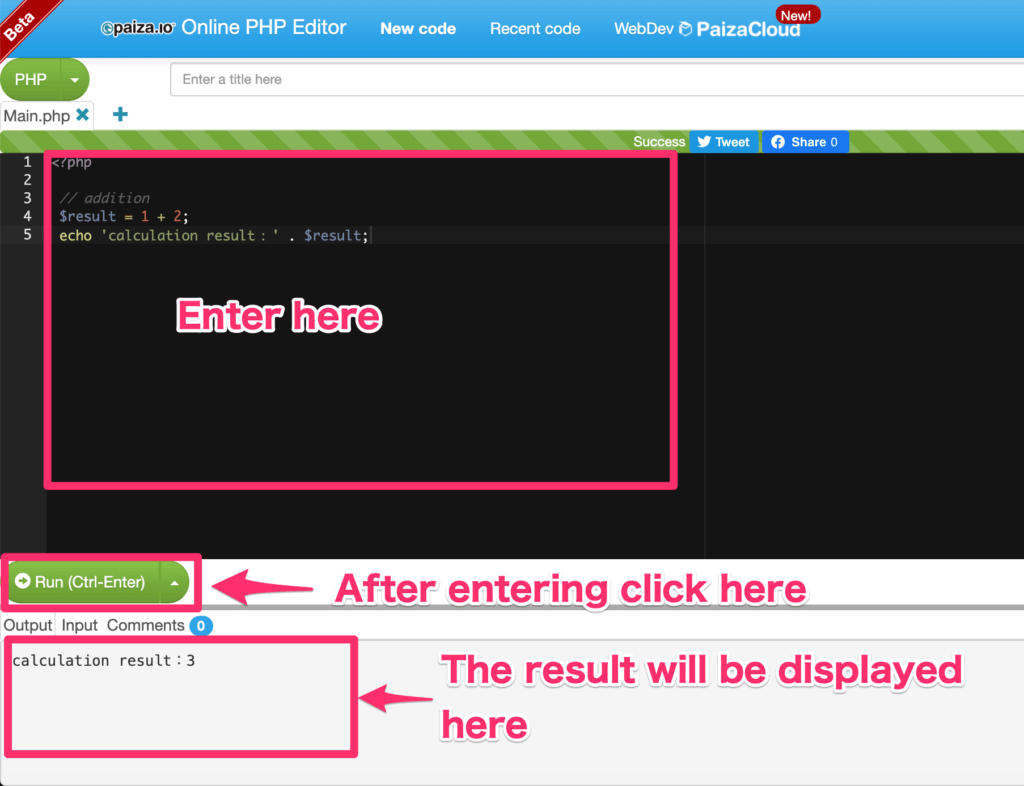Programming Lessons

# [PHP]Let’s Calculate![Programming for Kids and Above #3]Hello, I’m kat, the manager of ‘kat’s blog.

‘For the third installment of [Programming for Kids and Above], we will cover how to add, subtract, multiply, and divide in programming.

Calculations are the specialty of computers.

Even calculations that would take a long time for a human to solve, computers can do it in an instant.

## Why are computers fast?

Why is it that computers are so fast at doing calculations?

That is because, there is electricity flowing inside the computer, and that electricity is doing the calculations such as addition.

It is said that the speed of electricity is the same as the speed of light, and it is so fast that it cannot be seen by the naked eye.

This speed enables it to do calculations in an instant.

## What we will use this time

The programming language we will use this time

This time we will use the following programming language to write out a program.

・PHP

## Let’s calculate!

Let’s go ahead and write out a program.

This time, we will be programming on a website called ‘paiza.’

First, let’s display the website by clicking the link below.

Once the website is displayed, check to make sure that the button on the top left is showing as ‘PHP.’

If it hasn’t changed, choose ‘PHP.’Once you reach this stage, you are ready for the programming.

After writing in the source code, click the ‘Run (Ctrl-Enter)’ button like the following image.

Then the result will be displayed.

```<?php

\$result = 1 + 2;
echo 'calculation result：' . \$result;
```

Once you are done entering the necessary information, click the ‘Run’ button on the bottom left.If letters saying, ‘calculation result:3’ appear, then it is a success!

Let me give a brief explanation about the program.

~Explanation 1~

```<?php
```

This means the start of a PHP program.

It is a rule to always put ‘<?php’ at the beginning of a PHP program.

~Explanation 2~

```\$result = 1 + 2;
```

Here, we will be adding numbers.

‘\$result’ is something that is called a variable.

A variable is like a container, so you can put the value that is written after the ‘= (equal sign)’ into it.

Here, we put the result (‘3’) of ‘1+2’ into the ‘\$result’ variable (container).

✓ Point

In the case of PHP, there is a rule to always put a ‘\$ (dollar)’ sign at the beginning of a variable.

You can decide freely which letters come after the ‘\$’ sign.

Let’s try making variables with a name of your choice such as, ‘\$message,’ ‘\$color.’

~Explanation 3~

```echo 'calculation result：' . \$result;
```

Here, the calculation result is displayed on the screen.

The letters written after ‘echo,’ will be displayed on the screen.

The ‘.’ after ‘calculation result:’, is used to connect letters to letters (or variables).

Here it is connecting ‘calculation result:’ , and ‘\$result’.

Because the calculation result from earlier (“”3″”) is in the ‘\$result’, ‘calculation result:’ and ‘the value of \$result(“”3″”)’ are connected, and ‘calculation result:3’ is displayed on the screen.

## Subtraction

Next, let’s try some subtractions.

Enter the following into the source code input area.

```<?php

// subtract
\$result = 3 - 2;
echo 'calculation result：' . \$result;
```

Once you finish entering, click the ‘Run’ button on the bottom left like we did with the addition calculations.

If ‘calculation result:1’ is displayed, it is a success!

## Multiplication, Division

We follow the same steps for multiplication and division, but the symbols (operators) are different from what we are used to, so try to remember them.

■Operators for multiplication, division

Multiplication・・・’*’

Division・・・’/’

Now, let’s make a program like the one shown below.

```<?php

// multiply
\$result = 3 * 2;
echo 'multiplication calculation result：' . \$result . ',';

// divide
\$result = 4 / 2;
echo 'division calculation result：' . \$result;
```

It is a success if you click the ‘Run’ button and

‘multiplication calculation result:6,division calculation result:2’

is displayed.

Don’t just stick to simple calculations, but try calculations which are more difficult, such as combining addition and multiplication.

For example, what is the answer of 765 + 875 * 568 – 23 / 54?

A computer could give you the answer right away.

## Final thoughts

Calculation processes are written in pretty much all programs of machines.

We’ll be using them a lot in this lecture series, so please try to remember how to write them out.

Also, this time we only put one value in a variable, but next time I will explain how you can put multiple values into one variable, so please look forward to it!

See you next time!